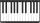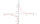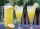# Fraction calculator

The calculator performs basic and advanced operations with fractions, expressions with fractions combined with integers, decimals, and mixed numbers. It also shows detailed step-by-step information about the fraction calculation procedure. Solve problems with two, three, or more fractions and numbers in one expression.

## Result:

### (2/3) : (1/4) = 8/3 = 2 2/3 ≅ 2.6666667

Spelled result in words is eight thirds (or two and two thirds).

### How do you solve fractions step by step?

1. Divide: 2/3 : 1/4 = 2/3 · 4/1 = 2 · 4/3 · 1 = 8/3
Dividing two fractions is the same as multiplying the first fraction by the reciprocal value of the second fraction. The first sub-step is to find the reciprocal (reverse the numerator and denominator, reciprocal of 1/4 is 4/1) of the second fraction. Next, multiply the two numerators. Then, multiply the two denominators. In the following intermediate step, the fraction result cannot be further simplified by canceling.
In other words - two thirds divided by one quarter = eight thirds.

#### Rules for expressions with fractions:

Fractions - simply use a forward slash between the numerator and denominator, i.e., for five-hundredths, enter 5/100. If you are using mixed numbers, be sure to leave a single space between the whole and fraction part.
The slash separates the numerator (number above a fraction line) and denominator (number below).

Mixed numerals (mixed fractions or mixed numbers) write as integer separated by one space and fraction i.e., 1 2/3 (having the same sign). An example of a negative mixed fraction: -5 1/2.
Because slash is both signs for fraction line and division, we recommended use colon (:) as the operator of division fractions i.e., 1/2 : 3.

Decimals (decimal numbers) enter with a decimal point . and they are automatically converted to fractions - i.e. 1.45.

The colon : and slash / is the symbol of division. Can be used to divide mixed numbers 1 2/3 : 4 3/8 or can be used for write complex fractions i.e. 1/2 : 1/3.
An asterisk * or × is the symbol for multiplication.
Plus + is addition, minus sign - is subtraction and ()[] is mathematical parentheses.
The exponentiation/power symbol is ^ - for example: (7/8-4/5)^2 = (7/8-4/5)2

#### Examples:

subtracting fractions: 2/3 - 1/2
multiplying fractions: 7/8 * 3/9
dividing Fractions: 1/2 : 3/4
exponentiation of fraction: 3/5^3
fractional exponents: 16 ^ 1/2
adding fractions and mixed numbers: 8/5 + 6 2/7
dividing integer and fraction: 5 ÷ 1/2
complex fractions: 5/8 : 2 2/3
decimal to fraction: 0.625
Fraction to Decimal: 1/4
Fraction to Percent: 1/8 %
comparing fractions: 1/4 2/3
multiplying a fraction by a whole number: 6 * 3/4
square root of a fraction: sqrt(1/16)
reducing or simplifying the fraction (simplification) - dividing the numerator and denominator of a fraction by the same non-zero number - equivalent fraction: 4/22
expression with brackets: 1/3 * (1/2 - 3 3/8)
compound fraction: 3/4 of 5/7
fractions multiple: 2/3 of 3/5
divide to find the quotient: 3/5 ÷ 2/3

The calculator follows well-known rules for order of operations. The most common mnemonics for remembering this order of operations are:
PEMDAS - Parentheses, Exponents, Multiplication, Division, Addition, Subtraction.
BEDMAS - Brackets, Exponents, Division, Multiplication, Addition, Subtraction
BODMAS - Brackets, Of or Order, Division, Multiplication, Addition, Subtraction.
GEMDAS - Grouping Symbols - brackets (){}, Exponents, Multiplication, Division, Addition, Subtraction.
Be careful, always do multiplication and division before addition and subtraction. Some operators (+ and -) and (* and /) has the same priority and then must evaluate from left to right.

## Fractions in word problems:

• Find the 11Find the quotient of 229.12 and 12.32
• TwelveTwelve students work on a week forestry brigade. One hundred spruces will receive x CZK, one hundred pine y CZK. How many receive each one students did in one day if they planted a total of 25,000 spruces per week and 30,000 pine trees? Express by express
• Youth trackYouth track from Hronská Dúbrava to Banská Štiavnica which announced cancellation attracted considerable media attention and public opposition, has cost 6.3 euro per capita and revenue 13 cents per capita. Calculate the size of subsidies to a trip group o
• Drinking waterA man drinks a keg of water in 40 days, and a woman drinks in 62 days. How many days do they consume a keg together?
• Larry 2Larry spends half of his workday teaching piano lessons. If he sees 6 students, each for the same amount of time, what fraction of his workday is spent with each student?
• Pie division5/8 of a pie divide 6 pieces. Each friend got 1/6.  What fraction  of the whole pie does each person receive?
• One half 2One half pizza will be divide among 3 pupils. Each pupil receive 1/6. Is it true or false?
• Quotient and divisionWhat is the quotient of 2/9 and 1/8?
• ReciprocalsWhich among the given reciprocal is correct a. 3/15x1/3= 1 b. 3/20x20/3=1 c. 7/14x7/7=1 d. 34/3x34/34=1
• AlmondsRudi has 4 cups of almonds. His trail mix recipe calls for 2/3 cup of almonds. How many batches of trail mix can he make?Six friends are sharing 8 cups of lemonade. If they share the lemonade equally, how many cups will each friend get?There are 2 2/3 pizzas. How many people are sharing when each has 2/3 of pizza?Lauren is tutoring students at the library on Saturday. If she is at the library for a total of 6 hours and she helps each student, one at a time, for three-fourths of an hour, how many students does she tutor?